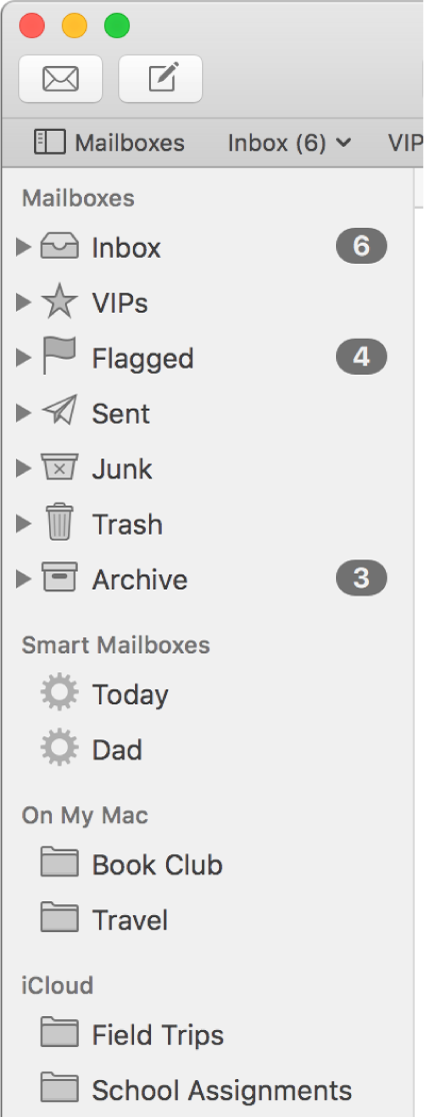127 views

# Badge in a listbox (x-platform)

1. 4 days ago

### Edwin v

May 15 Pre-Release Testers The Netherlands

I have been trying to add a badge to a cell in a listbox. But somehow it never really turns out the way I want.

In the forum I see lots of posts about the DockTile and some Mac declares.
The problem is that I need it to be cross platform.

My approach was to just create a global function that accepts a string to shown as a badge the text-size and maybe the colors I want. The function simply returns a Picture with a mask, that I can simply draw in any Graphics object. So, not limited to a Listbox... but also to a canvas.

Again... it never looks like the badges we all know and love...
So, in order not to re-invent the wheel... I turn to you guys. Any idea how this can be done?

2. ### Stephen T

May 15

I did something similar once before for some organisational badges/logo's.

I took a simple approach of collecting jpg images and adding them to a SQLite table as a blob, then drawing them in the listbox column when needed.

This was not cross platform though, I used and tested it in OSX only.

3. ### Edwin v

May 15 Pre-Release Testers The Netherlands
Edited 4 days ago

@Stephen T ...I took a simple approach of collecting jpg images and adding them to a SQLite table as a blob, then drawing them in the listbox column when needed...

Thanks for the quick reply.
But this is not what I was looking for. A badge is that little rounded rectangle with a number in it. Like the amount of messages in a mailbox in the Apple Mail app.

I think such a rounded rectangle can be generated with the text in it, and drawn in the cell in the DrawCellText event.4. ### Stephen T

May 15

Oh I see, I misunderstood your meaning of badge :)

I did create something similar a long time ago, and indeed it was a round rectangle, with digits at the appropriate position.

I think I used drawstring for the actual digits.

May 15
6. ### Dave S

May 15 San Diego, California USA

here is what I used in my TreeView control...

```Private Function Number_Badge(g as Graphics, value as integer) as picture
Dim old_size As Double
Dim new_size As Double
Dim s As String
Dim p As picture
Dim gg As Graphics
Dim x As Integer
Dim y As Integer
Dim w As Integer
Dim h As Integer
old_size=g.TextSize

new_size=Max(10,old_size/2)
s=Str(value)
g.TextSize=new_size
p=New picture(g.StringWidth(s+"WW"),g.TextHeight+6,32)
gg=p.Graphics
gg.TextSize=new_size
gg.bold=True
x=0
y=0
w=gg.Width-1
h=gg.Height-1
gg.ForeColor=&cffffff

gg.FillRect 0,0,gg.Width,gg.Height

gg.ForeColor=&c3a3a3a
If zCountStyle=False Then
gg.FillRoundRect x,y,w,h,h,h
Else
gg.FillRect x,y,w,h
End If
x=x+1
y=y+1
w=w-2
h=h-2
gg.ForeColor=&cfefefe
If zCountStyle=False Then
gg.FillRoundRect x,y,w,h,h,h
Else
gg.FillRect x,y,w,h
End If
x=x+1
y=y+1
w=w-2
h=h-2
gg.ForeColor=zBadgeColor
If zCountStyle=False Then
gg.FillRoundRect x,y,w,h,h,h
Else
gg.FillRect x,y,w,h
End If
w=gg.Width-1
h=gg.Height-1
gg.ForeColor=&cfefefe
x=1+(w-gg.StringWidth(s))\2
y=gg.TextAscent+(h-gg.TextHeight)/2
gg.drawstring s,x,y
g.TextSize=old_size
p.Transparent=1
Return p

End Function```
7. ### Markus W

May 15 Pre-Release Testers, Xojo Pro #JeSuisHuman Germany, Heidelb...

@Dave S here is what I used in my TreeView control...

```Private Function Number_Badge(g as Graphics, value as integer) as picture […] End Function```

Why do you hate comments so much ? ;)

8. ### Edwin v

May 15 Pre-Release Testers The Netherlands

@Dave S here is what I used in my TreeView control...

That's awesome... But... I think I found the reason why my badges looked bad... this is what I came up with now:The code:

```Public Function makeBadge(value as String, textSize as Integer = 10, backColor as color = &c454645, textColor as color = &cF5F5F5) as Picture
Dim t as String = value.Trim
if t = "" then Return nil

if textSize < 8 then textSize = 8

Dim p as Picture
Dim g as Graphics

Const kBold as Boolean = true
Const kUnderline as Boolean = False
Const kItalic as Boolean = False
Dim kPaddingH as Integer = textSize * 0.55
Dim kPaddingV as Integer = textSize * 0.15

// First get the size of the final badge
p = new Picture(10, 10, 32)
g = p.Graphics

g.TextSize = textSize
g.Bold = kBold
g.Italic = kItalic
g.Underline = kUnderline

Dim tw, th as Integer
tw = g.StringWidth( t )
th = g.StringHeight( t, tw + 1 )

Dim width, height as Integer
width = tw + ( kPaddingH * 2 )
height = th + ( kPaddingV * 2 )

// Make badge picture object
Dim badge as new Picture(width, height, 32)
g = badge.Graphics

// Fill with background color
g.ForeColor = backColor
g.FillRect 0, 0, width, height

// Set text properties
g.TextSize = textSize
g.Bold = kBold
g.Italic = kItalic
g.Underline = kUnderline
g.ForeColor = textColor

// Get text position
Dim x, y as Integer
x = kPaddingH
y = kPaddingV

// Draw the badge text
g.DrawString t, x, y + g.TextAscent

// Prepare the mask

Dim mask as new Picture(width, height, 32 )
g = mask.Graphics

g.ForeColor = &cFFFFFF
g.FillRect 0, 0, width, height

Dim radius as Integer = min( width, height )

g.ForeColor = &c000000
g.FillRoundRect 0, 0, width, height, radius, radius

// Add mask to the badge Picture and return it
badge.Mask = mask
Return badge

End Function
```or Sign Up to reply!